﻿ Optimal Controller for Single Area Load Frequency Control via LQR and Legendre Wavelet Function

### Optimal Controller for Single Area Load Frequency Control via LQR and Legendre Wavelet FunctionOPEN ACCESSPEER-REVIEWED

## Optimal Controller for Single Area Load Frequency Control via LQR and Legendre Wavelet Function

Hatem Elaydi1,, Mohammed Wadi2

1Electrical Engineering Department, Islamic University of Gaza, Gaza, Palestine

2Electrical Engineering Department, Yildiz Technical University, Istanbul, Turkey

### Abstract

Load frequency control (LFC) is an important tool to insure the stability and reliability of power systems. Nowadays power demand is increasing continuously and the biggest challenge is to provide uninterrupted electrical energy to consumers under changing conditions. For satisfactory operation, the frequency of power system should be kept near constant value or within standard value; in addition to that an uninterrupted power should continue to flow despite the variations of load in different areas. Many techniques have been proposed to guarantee the uninterrupted flow of power and the constant value of frequency and to overcome any deviations. In this study, optimal control based on Legendre wavelet function and linear quadratic regulator (LQR) techniques are applied to single area power system in order to approximate the gain of controller to overcome the fluctuations of frequency in electrical power systems. This paper models a single area power system with optimal control strategies based on LQR and Legendre wavelet transform and presents simulation results using Simulink/MATLAB. The paper also compares the performance of the LQR and Legendre wavelet transform controllers. A numerical example is solved in order to demonstrate the effectiveness of the proposed technique.

### At a glance: Figures

123
Prev Next

• Elaydi, Hatem, and Mohammed Wadi. "Optimal Controller for Single Area Load Frequency Control via LQR and Legendre Wavelet Function." Journal of Automation and Control 3.2 (2015): 43-47.
• Elaydi, H. , & Wadi, M. (2015). Optimal Controller for Single Area Load Frequency Control via LQR and Legendre Wavelet Function. Journal of Automation and Control, 3(2), 43-47.
• Elaydi, Hatem, and Mohammed Wadi. "Optimal Controller for Single Area Load Frequency Control via LQR and Legendre Wavelet Function." Journal of Automation and Control 3, no. 2 (2015): 43-47.

 Import into BibTeX Import into EndNote Import into RefMan Import into RefWorks

### 1. Introduction

During the last three decades, power generation systems have become more complex and largely interconnected and they required automatic generation control (AGC) in order to keep the systems operating safely [1, 2, 3, 4]. Automatic generation control is also referred to as load frequency control (LFC). The frequency of a power system is affected by changes in real power, while the voltage magnitude is dependent on changes in reactive power. Thus, active and reactive powers can be controlled separately to meet different criteria. In an isolated power system, the main objective of an LFC is to maintain a balance of a power system by maintaining the frequency within an acceptable tolerance around a nominal system frequency. The reliability, security and stability are three required properties in order to balance any power system efficiently. Reliability is defined as the ability of the system to adequately supply a near continuous electric services with few interruptions over an extended period of time, security is defined as the resistance of a power system to disturbances to supply consumers with power without discontinuity and indicates the level of robustness of the power system to a disturbance at any instant in time, while stability is defined as the ability of the power system to return to an equilibrium operating state by keeping frequency and voltage to be within acceptable standard levels .

Typically, the power output of the generators and the difference between the instant loads change the nominal frequency. If the amount of production is more/less than demanded power, the speed of generators increases/decreases; hence, frequency increases/decreases. When deviation occurs, frequency is brought to a desired level by controlling the speed of generators. Thus, generated power and frequency in the system should be adjusted according to the consumption; this process is called LFC model.

In an isolated power system, frequency control is considered a challenging issue due to low system inertia, nonlinear effects of physical components such as governor dead zone and generation rate constraints. Furthermore, any mismatch between generation and demand deviates the system frequency from scheduled values. High frequency deviation may lead to system collapse. In order to maintain constant nominal frequency, an accurate and fast acting controller such as optimal controller is required . This study proposes an optimal control strategy based on Legendre wavelet function to control load frequency. The optimal control is based on LQR control and utilizes the direct method to parameterize the cost function and constraints using Legendre wavelet functions. The optimal control strategy is applied to a single area power system with a load frequency control model in order to bring the steady-state frequency error to zero.

### 2. Load Frequency Control Model of Single Area Power Systems

In large electrical power system, nominal frequency depends significantly on the balance of produced and consumed active power. When active power imbalance occurs in any part of the system, it results in changes in the frequency of the entire system. Industrial loads connected to electrical power system are very sensitive to the quality of electrical energy, mainly the frequency component. Thus, the steady-state frequency error in the system must stay within acceptable values in order to keep the balance . Figure 1 shows the relationship between system frequency and load which is inversely proportional. Possible increase in the load reduces the nominal frequency of the system. This alternation in frequency is sensed by a regulator in the primary control loop; subsequently, the rotational speed of the turbine is increased leading to an increase in the produced power. Load frequency control is accomplished using two stages: primary control and secondary control. Figure 2 illustrates the main components of LFC for single area power system which can be represented in a matrix form as shown in equation (1) [8, 9].(1)

### 3. Linear Quadratic Optimal Problem

Optimal control is an important science that deals with nonlinear optimal control problem (OCP) and the main objective of optimal control is to find an optimal controller that can be applied to a nonlinear system as well as to a linear and time varying system to minimize a certain cost function within the system's physical constraints. In other words, the main task of an optimal controller is to transfer the system from its initial state to the final state with minimum cost function . Generally, optimal control can be classified as direct and indirect methods. Indirect methods are based on converting OCP into two-point boundary value problem (TPBVP), then solving the last one by Euler Lagrange technique or Hamilton-Jacobi-Bellman equation (HJBE). Direct methods can be implemented using discretization or parameterization methods. Mathematically, the optimal control problem can be considered as finding the optimal controller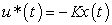that minimize a performance index such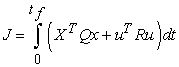(2)

Subject to linear constraints and initial condition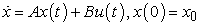(3)

WhereA,B are n×n and n×m constant matrices respectively. Q is an n×n positive semidefinite matrix and R is an m×m positive definite matrix.

3.1. Optimal Control via Legendre Wavelet Function

One of the most famous indirect optimal control techniques that are used recently to approximate linear time invariant (LTI), linear time varying (LTV) and nonlinear systems are wavelet functions . Legendre wavelet function has been used to approximate different systems within the subspace of trained initial condition and is considered a powerful function to give accurate approximation. Legendre wavelet function is defined such as .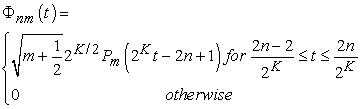Where Pm is the Legendre polynomial of order m; n refers to the section number, n=1,2,…2K-1 ; K is the scaling parameter and can assume any positive integer and t∈[0,1]. Legendre polynomial can be defined as in  as follows:(5)

State and control variables can be approximated using Legendre scaling function as follows: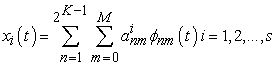(6)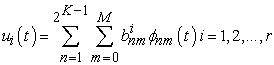(7)

Here, the quadratic form of optimal problem is constructed and may be solved easily using Matlab .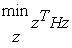(8)

Subject to equality constraints(9)

whereThe optimal vector z* can be also calculated by the standard quadratic programming method  as follows:(10)
3.3. Optimal Control via LQR

The linear quadratic regulator (LQR) is used to design optimal controllers for linear systems with quadratic performance index. LQR can be realized by finding optimal closed-loop state feedback control. In this article, LQR is applied to determine an appropriate state feedback gain along with the minimum variance method to design an LFC for single area power system. LQR can achieve good performance with minimum cost and can be easily designed using MATLAB. On the other hand, LQR has many limitations such as: it requires full state feedback, its function is only to guarantee stability, and it uses quadratic indices. This paper proposes a Legendre wavelet function to design optimal controller and compares its performance with an LQR controller.

### 4. Simulation Results

A comparison between an optimal controller based on Legendre wavelet function and an LQR control is made with the purpose of controlling LFC. The comparison is made in terms of performance with respect to frequency deviation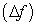, settling time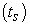and steady state error. The parameters of the numerical example which is solved using Simulink/Matlab and programming subscripts are shown in Table 1. In addition, the solution of an example consists of three scenarios: the first one contains no controller, the second solution uses an LQR control, and the final scenario uses an optimal controller based on Legendre wavelet function.

#### Table 1. Parameters of single area power station 

The state space system and performance index of above example are respectively as follow :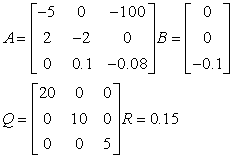(11)(12)
4.1. First Scenario

In the first scenario, a block diagram of LFC is constructed using Simulink and solved without using any controller with the power increasing by 200 MW (0.2 p.u). Figure 3 and Figure 4 show the Simulink block diagram of LFC and the frequency deviation respectively; while Table 2 summaries the obtained results.

4.2. Second Scenario

In the second scenario, an LQR optimal controller is designed to approximate the state feedback gain vector. The LQR controller is designed using M-file and Simulink. The block diagram and the frequency deviation response are shown in Figures 5 and 6 respectively. Table 3 summarizes the obtained results. However, the optimal gain vector k=[6.41 1.10 -112.60] is obtained at end of simulation.

#### Table 3. Results of second scenario for LFC using LQR

4.3. Third Scenario

A complete reference should contain the name(s) of the author(s) and/or editor(s), the title of the article, the name of the book or conference proceedings where appropriate, and bibliographic information about the article such as the name of the publisher, the city of publication, and the page numbers. The basic concept is that the reference should be sufficiently complete so that the reader could readily find the reference and can judge the authority and objectivity of the reference.

In the third scenario, an optimal controller based on Legendre wavelet function is obtained using the approximation techniques to design the feedback gain vector K by converting the problem into a quadratic form that can be easily solved using Matlab. Furthermore, approximation via Legendre function is based on two factors: the order of Legendre polynomial (M) and the positive integer scaling parameter (K) which increases the accuracy of approximation proportionally. In this paper, the problem is solved for M=4, K=2 and M=6, K=2. Figures 7 and 8 illustrate the response of LFC for M=4 and M=6 respectively; while Table 4 summaries the obtained results.

#### Table 4. Results of third scenario for LFC using Legendre function

As seen from Table 5, we can conclude that the optimal controller via Legendre wavelet function provides better performance and response than LQR. Both controllers offer the same settling time, but the Legendre wavelet function controller gives better performance in terms of the frequency deviation and steady state errorby fifteen times less than LQR.

Figure 8. Frequency deviation of third scenario for LFC via Legendre function at M=6, K=2

### 5. Conclusion

In this paper, a new optimal controller via Legendre wavelet function was proposed. A comparison was made between an LQR optimal controller and an optimal controller based on Legendre wavelet function in terms of performance aspect in single areas power systems. Simulation results showed that the optimal controller based on Legendre wavelet function approximation method outperformed the LQR controller in terms of less frequency deviation and steady state error; while both had the same settling time. A numerical example demonstrated the effectiveness of the proposed optimal control via Legendre wavelets function over LQR control.

### References

  Benjamin NN, Chan WC., “Variable structure control of electric power generation,” IEEE Transaction on Power Apparatus & Systems, PAS-101 (2). 376-380, 1982.In article CrossRef  Pan CT, Liaw CM., “An adaptive controller for power system load-frequency control,” IEEE Transactions on Power Systems, 4 (1). 122-128, 1989.In article CrossRef  Kothari ML, Nanda J, Kothari DP, Das D., “Discrete-mode automatic generation control of a two-area reheat thermal system with new area control error,” IEEE Transaction on Power Systems, 4 (2). 730-738, 1989.In article CrossRef  Wang Y, Zhou Y R, Wen C., “New robust adaptive load-frequency control with system parametric uncertainties,” IEEE Transactions on Generation, Transmission & Distribution, 141 (3). 184-190, 1994.In article CrossRef  Gillian R. L., “Frequency control on an island power system with evolving plant mix,” PhD, National University of Ireland, Dublin, Ireland, 2005.In article PubMed  Usman A, Divakar B., “Simulation study of load frequency control of single and two area systems,” In IEEE 2012 Global Humanitarian Technology Conference, 214-219, Oct 2012.In article CrossRef  Demiroren A, Yeşil, E., “Automatic generation control with fuzzy logic controllers in the power system including SMES units,” Electrical Power & Energy Systems, 26. 291-305, 2004.In article CrossRef  George G, Jeong W L., “Analysis of load frequency control performance assessment criteria,” IEEE Transaction on Power Systems, 16 (3). 520-525, 2001.In article CrossRef  Adnan B, Ebenezer JA., “Load frequency control with fuzzy logic controller considering nonlinearities and boiler dynamics,” ACSE, l8 (3).15-20, 2009.In article  Gozde, H, Taplamacıoglu, MC, Kocaarslan, I and Senol, MA., “Particle swarm optimization based pi-controller design to load-frequency control of a two area reheat thermal power system,” Journal of Thermal Sciences & Technologies, 30 (1). 13-21, 2010.In article  Saadat H., Power System Analysis, 3rd ed. New York, NY, USA: McGraw-Hill, 2002.In article  Jaddu H., “Optimal control of time-varying linear systems using wavelets,” PhD, Japan Advanced Institute of Science and Technology, Ishikawa, Japan, 2006.In article  Wadi M., “Solving quadratic optimal control problems using Legendre scaling function and iterative technique,” MSc, Islamic University of Gaza, Gaza, Palestine, 2011.In article PubMed